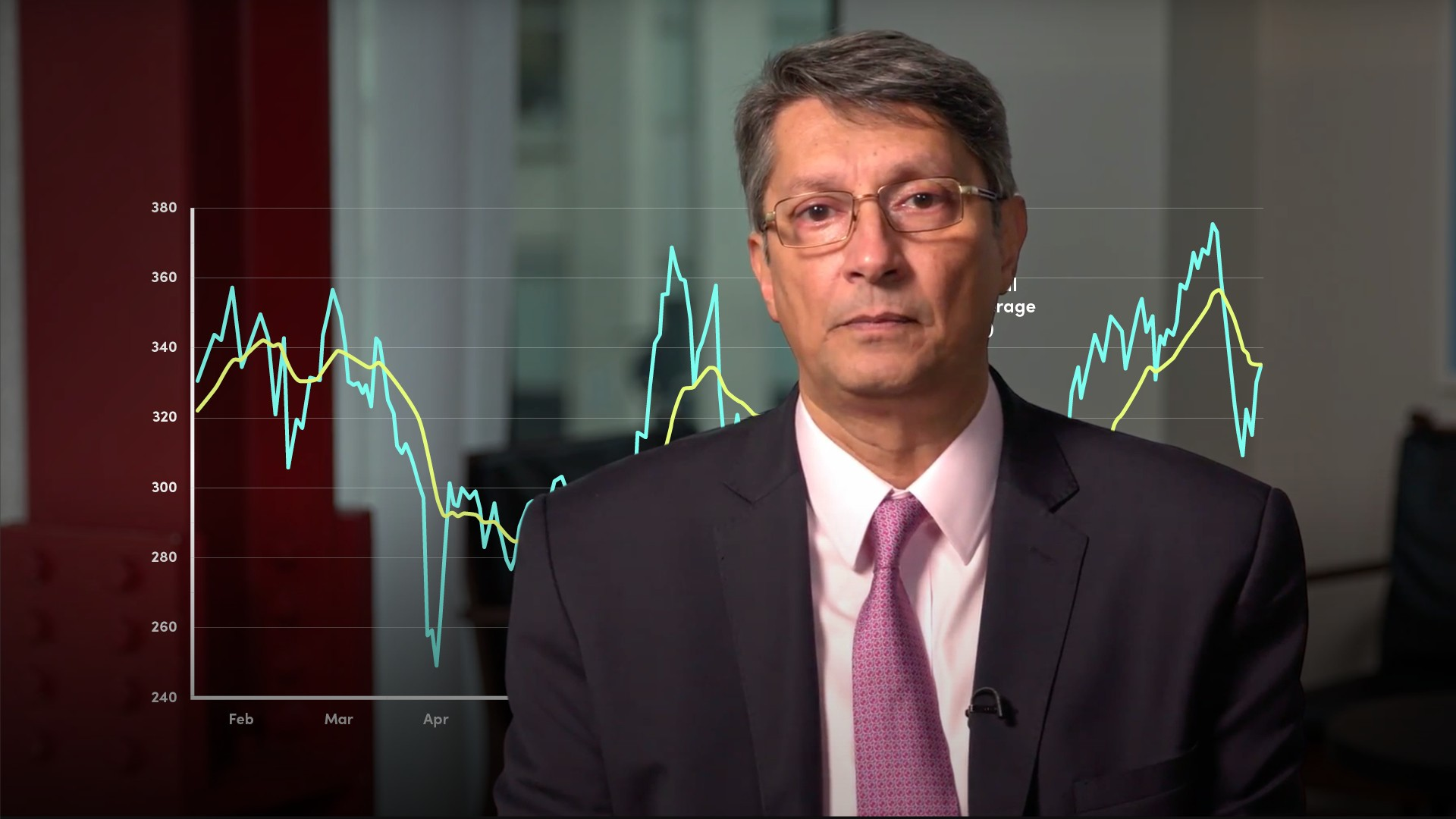# Moving Averages (2/2): Weighted and Exponential Moving Averages### Abdulla Javeri

In the previous video, Abdulla covered simple or arithmetic moving averages. Here, Abdulla explains a similar concept: the weighted and exponential moving averages. He answers the questions: what are they, how are they calculated and how are they used?

Financial Modelling & Mathematics